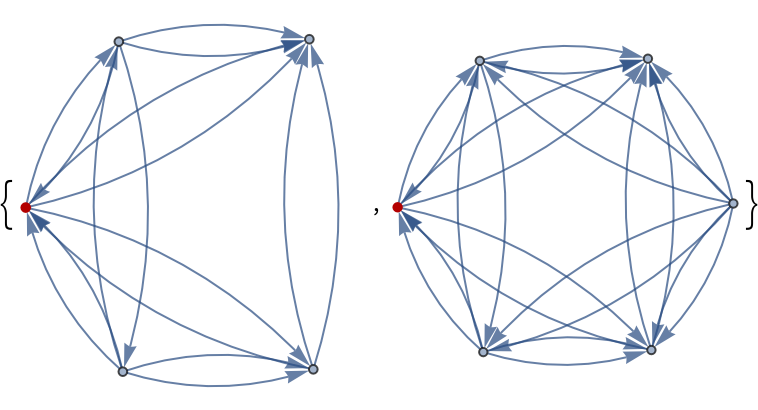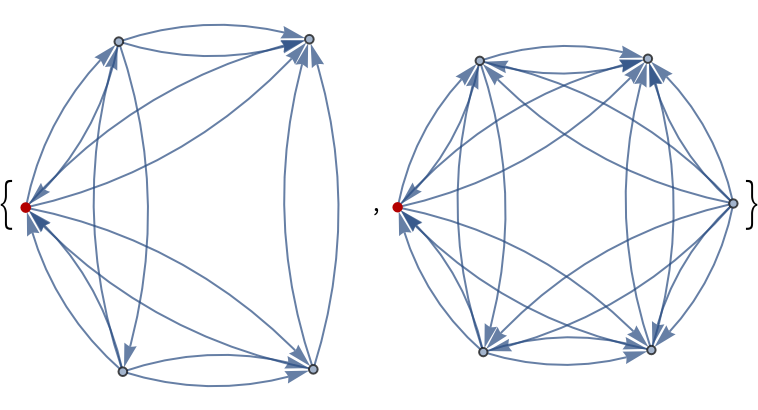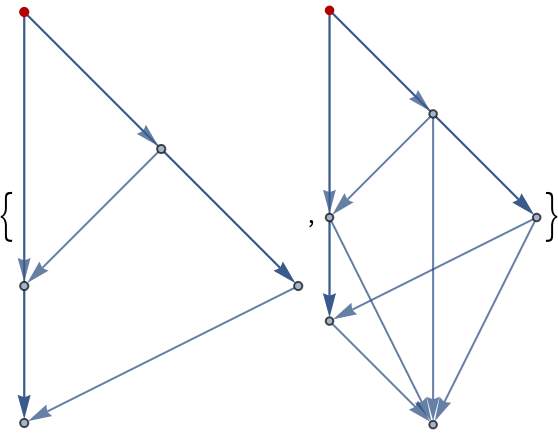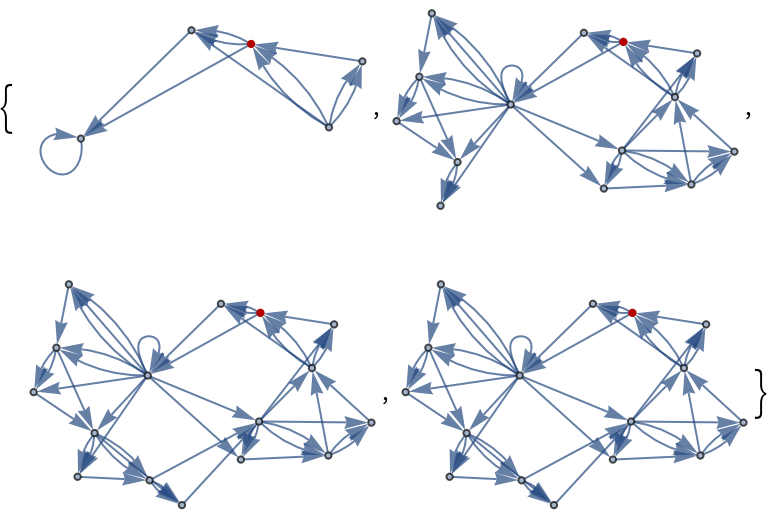Function Repository Resource:

# HypergraphNeighborhoods

Enumerate all the neighborhoods of a hypergraph up to a given distance

Contributed by: Stephen Wolfram
 ResourceFunction["HypergraphNeighborhoods"][hg,v,r] gives all the neighborhoods of the hypergraph hg up to a distance r from v. ResourceFunction["HypergraphNeighborhoods"][hg,r] gives all the neighborhoods of the hypergraph hg up to a distance r from the middle vertex in a sorted vertex list.

## Details and Options

ResourceFunction["HypergraphNeighborhoods"] converts hyperedges to single edges, so the hyperedge {1,2,3} is converted to {{1,2},{2,3},{1,3}}. A length-n hyperedge is converted into (n-1)! edges.
The distance-k neighborhood of a vertex is the set of vertices at graph distance k.

## Examples

### Basic Examples (2)

With the face indices of an octahedron as a hypergraph, find the first two neighborhoods of vertex 3:

 In:=Out=Since vertex 3 is the middle-low vertex in (1,2,3,4,5,6), the two-argument form of the function returns the same result:

 In:=Out=### Scope (2)

The edges of an octahedral graph give different neighborhoods:

 In:=Out=The first two neighborhoods of vertex 8 in a more complex hypergraph:

 In:=Out=## Version History

• 2.0.0 – 14 April 2020
• 1.0.0 – 18 February 2020